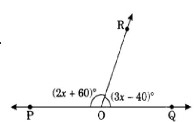"
">

# Find the value of $x$ in the given figure."

Given:

In the given figure, $(2x+60)^o$ and $(3x-40)^o$ form a linear pair.

To do:

We have to find the value of $x$.

Solution:

We know that,

Sum of the angles in a linear pair is $180^o$.

$(2x+60)^o+(3x-40)^o=180^o$

$5x+20^o=180^o$

$5x=180^o-20^o$

$5x=160^o$

$x=\frac{160^o}{5}$

$x=32^o$

The value of $x$ is $32^o$.

Updated on: 10-Oct-2022

33 Views